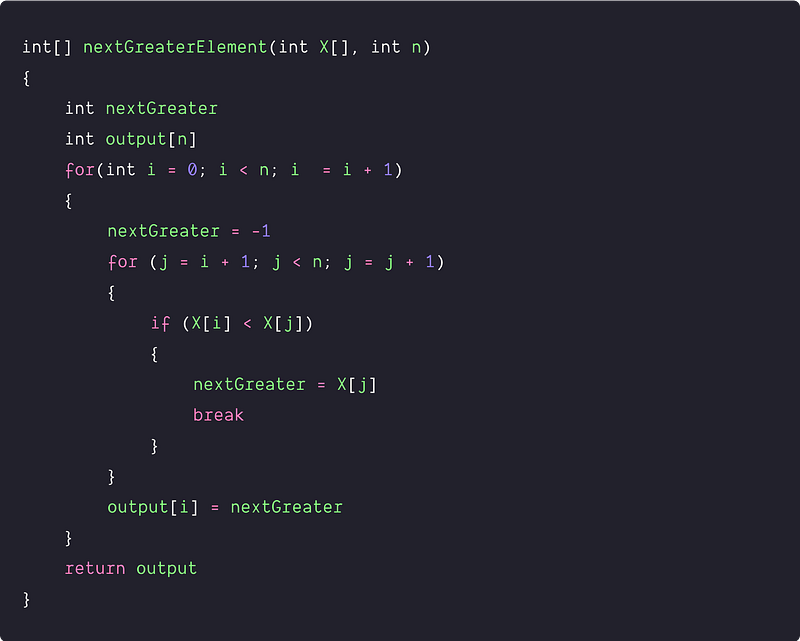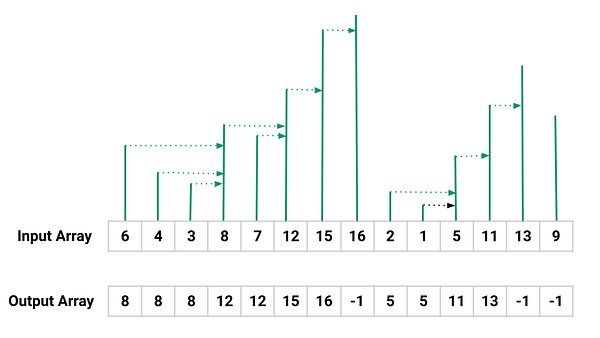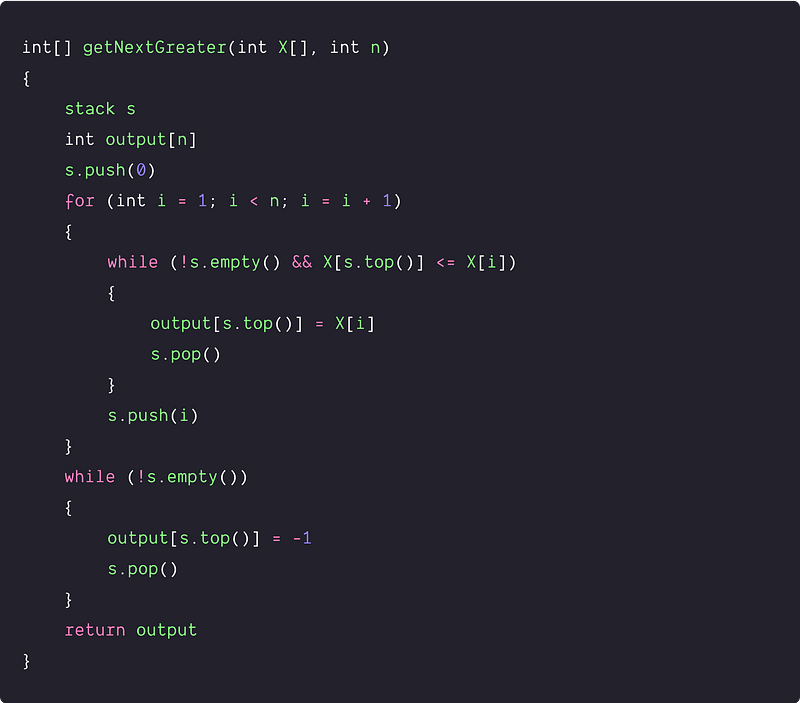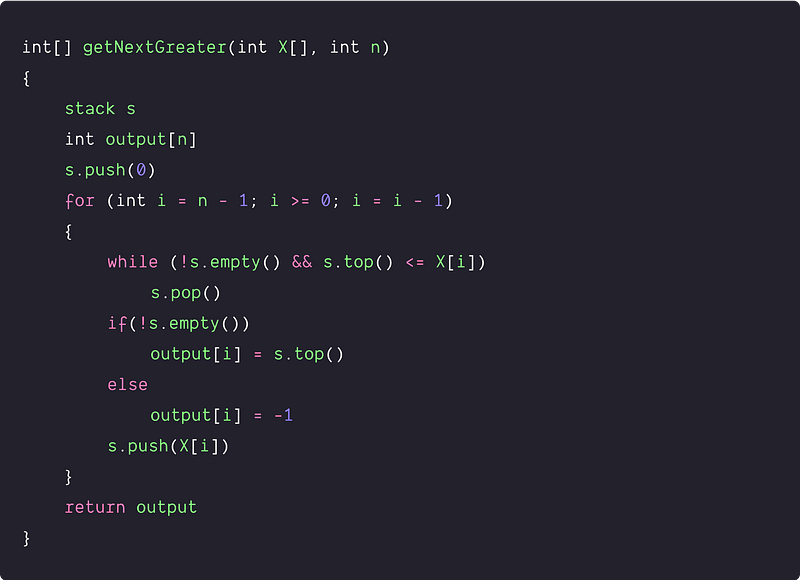# Find next greater elements in an array

Key takeaway: An excellent problem to learn problem-solving using stack.

### Let’s understand the problem

Given an array, find the Next Greater Element for every element. The Next greater Element for an element is the first greater element on the right side of the array. Elements for which no greater element exist, consider the next greater element as -1.

Examples

Input : A[] = [3, 2, 8, 7, 6, 17, 12], Output: [5, 8, 9, 17, 17, -1, -1]

Explanation: Traversing through the array for each element, we find the next greater element on its right side i.e: NGE of 3 is 8, NGE of 2 is 8, NGE of 8 is 9, NGE of 8 is 17, and so on.

Input : A[] = [4, 5, 2, 25, 10], Output: [5, 25, 25, -1, -1]

### Discussed solution approaches

• A brute force  approach using nested loops
• An efficient solution using stack : traversing from left to right
• An efficient solution using stack : traversing from right to left

### Brute force using nested loops

Solution Idea and Steps

The basic idea would be to traverse the array and find the next greater element for each element on the right side. We can simply use two nested loops for this.

• We run outer loop from i = 0 to n-1 to access each element X[i] in the array and run inner loop from j = i + 1 to n-1 to access all other elements on the right side of the current element X[i]. Inside the inner loop, we find the next greater element of the element X[i].
• We use an extra array output[n] to store the next greater element for each element. When we find the next greater element for an element we break from the inner loop and store it at output[i].
• Otherwise, the inner loop will go till the end and we didn't find an element that is greater than the element X[i]. In this situation, we store -1 at output[i].
• By end of the loop, we return the array output[i].

Solution PseudocodeTime and space complexity analysis

Here outer loop is runing n times and for each iteration of the outer loop, the inner loop is running n-i-1 times. So total number of loop iteration = n - 1 + n - 2 + .... + 1 + 1 = n(n-1)/2 + 1 = O(n^2). We are performing O(1) operation at each loop iteration loop, so time complexity = O(1) * O(n^2) = O(n^2)

Space complexity = O(1), as we are using a constant amount of memory to find and store the next greater element in the output[] array. Here output[] array will not be the part of the space complexity because it is the part of the output in the given problem. Think!

### An efficient solution using stack : traversing from left to right

Solution Idea

Now the critical question is: how do we improve the time complexity. Is there some pattern in the problem which could help us to find an effcient solution? Let's think!If we look at the input array from left to right, then there are two observations:

• Case 1: decreasing order sequence

In this case, the first next greater value is the next greater element of all the values in the decreasing sequence. For example, 6, 4, and 3 are in decreasing order, so the next greater element would be 8 which is the first next greater value. Similarly, 8, 7, and 2, 1 are in decreasing order, so there next greater element would be 12 and 5.

• Case 2: increasing order sequence

In this case, all the next value is greater than its previous value, so the adjacent element of each element is the next greater element. For example, 12, 15, and 16 are in increasing order, so the next greater element of 12 would be 15, and the next greater element of 15 would be 16. Similarly, 5, 11, and 13 are in increasing order, so the next greater element of 5 would be 11, and the next greater element of 11 would be 13.

So one idea is: scan from left to right and when we find the first element which is greater than the previous element we stop and save it as the next greater element of the previous element. Even this greater element can be the next greater element of some previous consecutive elements. The critical question is: how do we identify such previous elements? The idea is simple: we need a mechanism to store the occurrences of the previous elements i.e. we can use the stack for this purpose!

Solution Steps

• We create an empty stack s.
• We also declare an output[n] array to store the next greater elements.
• Now we traverse the array from the left to right and push the element into the stack till the element at the top of the stack is greater than the current element. (Think!)
• Now current element is greater than the top element in the stack. So we keep popping elements in a loop till the top of the stack is smaller than the current element or the stack becomes empty. During this process, we set the current element as the next greater element of all the popped elements (Think!).
• Now we push the current element into the stack and repeat the above process.
• Once all the elements are processed in the array but the stack may not be empty. So remaining elements in the stack don’t encounter any greatest element. So we pop the element from the stack and update -1 as their next greater element.
• By the end of the above process, we return the output[] array.

Note: we are pushing indexes into the stack instead of the actual elements.

Solution PseudocodeTime and space complexity analysis

Every element is pushed and popped at most once from the stack. So time complexity = O(n). Space complexity in the worst case = O(n), for the stack.

### An efficient solution using stack : traversing from right to left

Solution Idea

Here the idea is similar to the above approach but we process elements from right to left in the array.

1. We create an empty stack s.
2. We also declare an output[n] array to store the next greater element.
3. Now we run a loop from i = n - 1 to 0 to access each array element to find the next greater element.

• We push elements into the stack when the stack is empty or s.top() > X[i]. In other words, the stack will maintain the order of elements in decreasing order. Think!
• Now when we find s.top() <= X[i] and stack is not empty, we continuously pop elements from the stack.
• After the end of the above process, if the stack is not empty then the next greater element of X[i] will be present at the top of the stack and we update it with value s.top().
• If the stack is empty, then we update -1 as the next greater element of X[i].
4. By the end of the above process, we return the output[] array.

Note: Here we are pushing actual elements into the stack.

Solution PseudocodeTime and space complexity analysis

Every element is pushed and popped at most once to the stack. So time complexity = O(n). Space complexity in the worst case = O(n), for the stack.

### Critical ideas to think!

• Can we solve this problem using some other approaches?
• Can we try to use other data structures other than the stack? If yes, then which one?
• What will be the worst and best-case scenario of the space complexity in the stack-based approaches?
• How do we modify the above approaches if the given array is circular?

### Comparison of time and space complexities

• Using nested loops: Time = O(n^2), Space = O(1)
• Stack  solution traversing from left to right: Time = O(n), Space = O(n)
• Stack  solution traversing from right to left: Time = O(n), Space = O(n)

### Suggested coding questions to practice

Enjoy learning, Enjoy thinking, Enjoy algorithms!

Get well-designed application and interview centirc content on ds-algorithms, machine learning, system design and oops. Content will be delivered weekly.